# Electric potential and capacitance Electrostatic potential Potential due

• Slides: 37Electric potential and capacitanceElectrostatic potential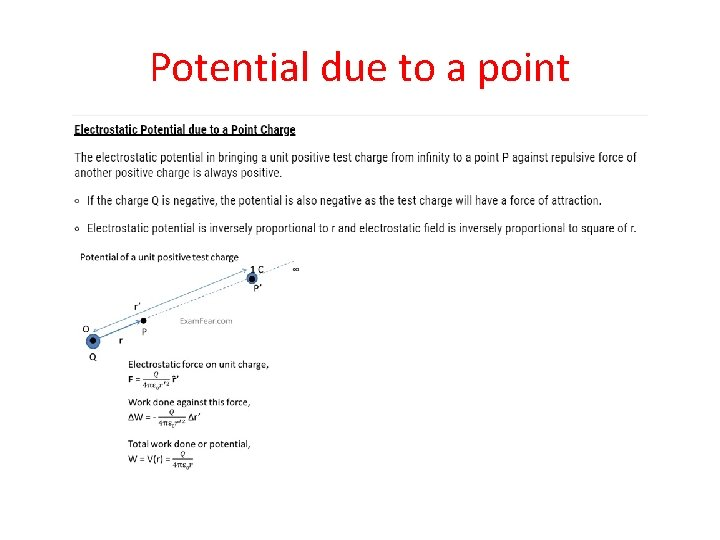Potential due to a point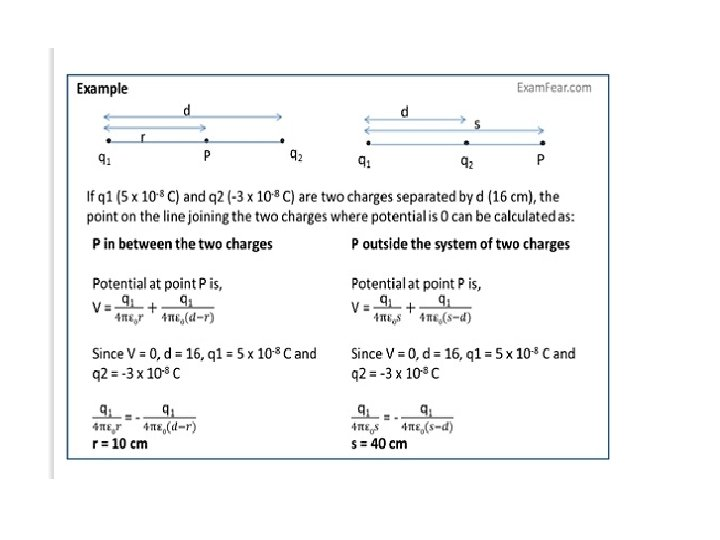Potential due to a dipole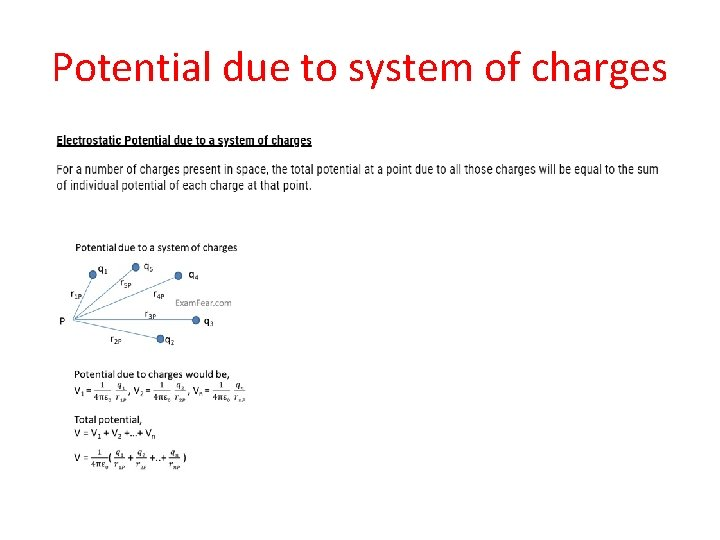Potential due to system of charges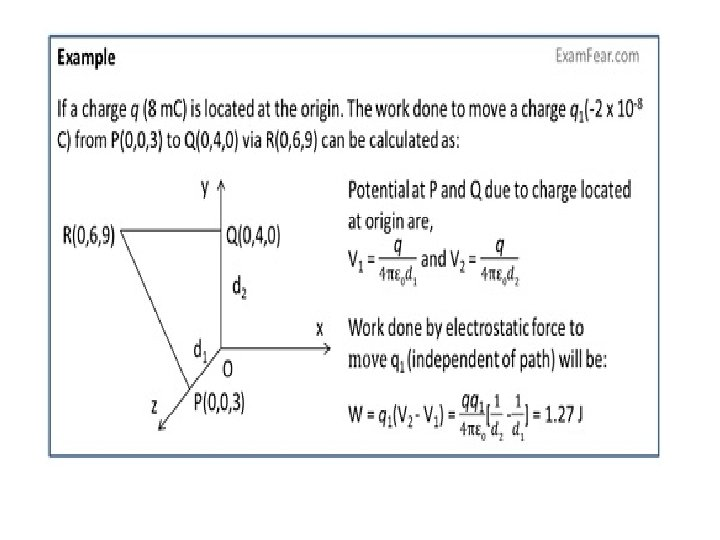Equipotential surfaces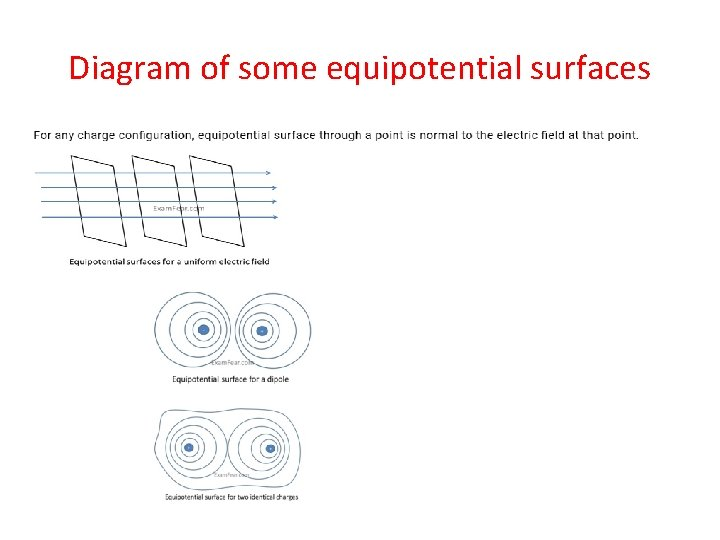Diagram of some equipotential surfacesRelation between ‘E’ and ‘V’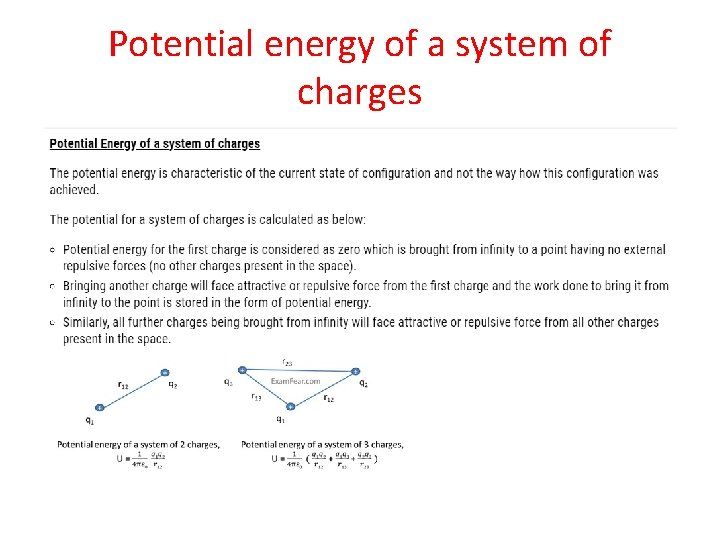Potential energy of a system of charges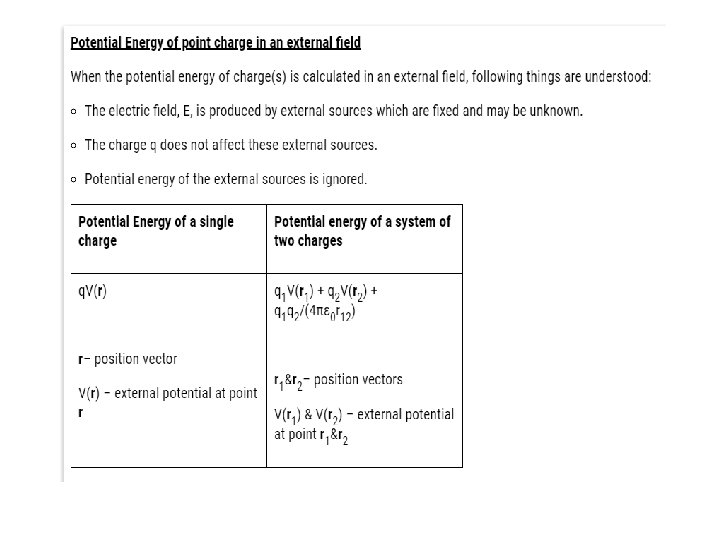P. E. of a dipole in an external field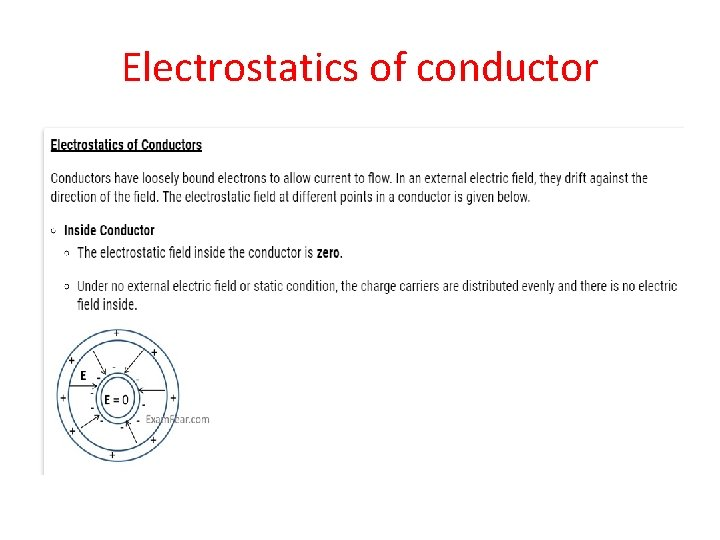Electrostatics of conductorElectrostatics of conductor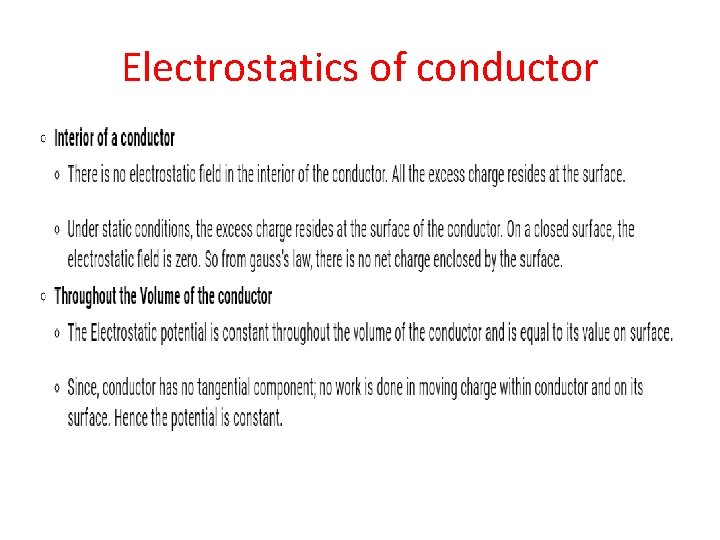Electrostatics of conductor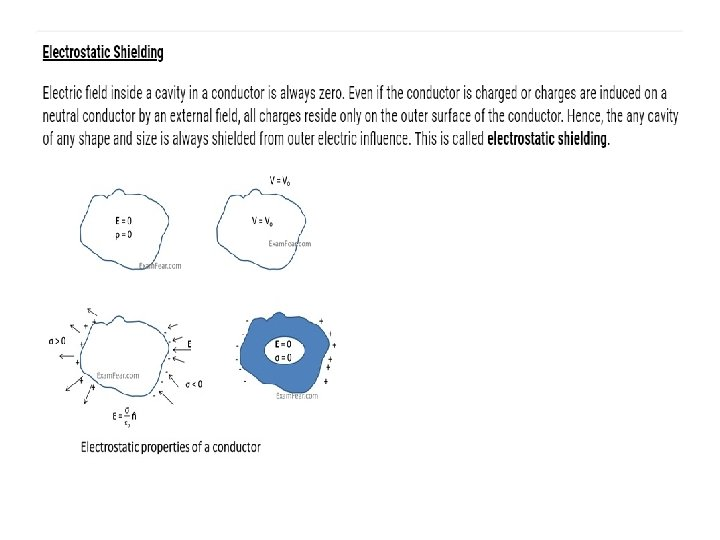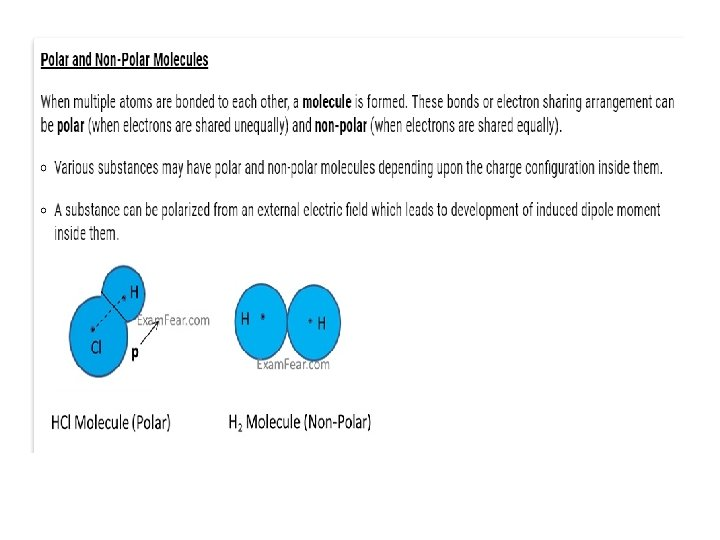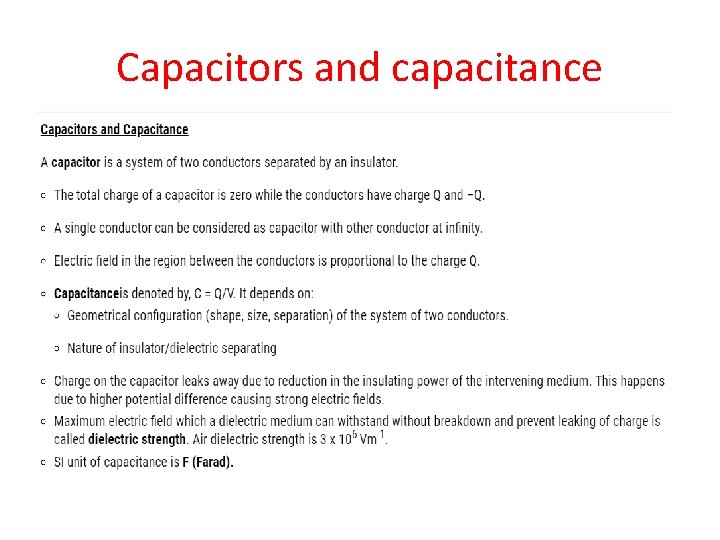Capacitors and capacitance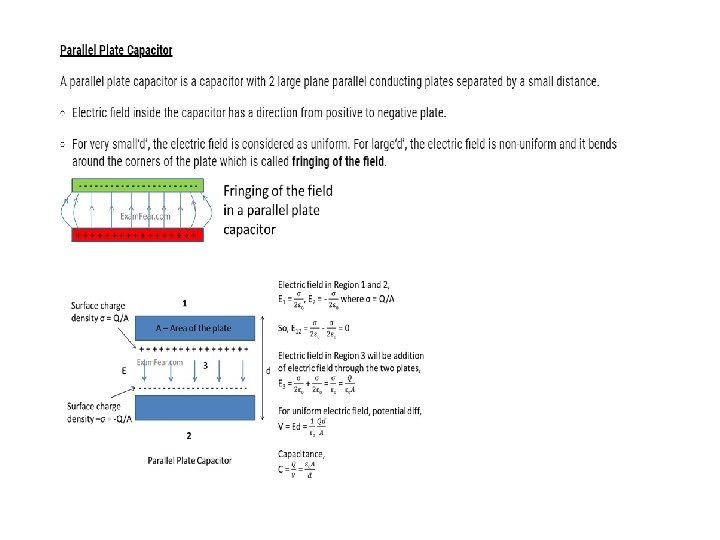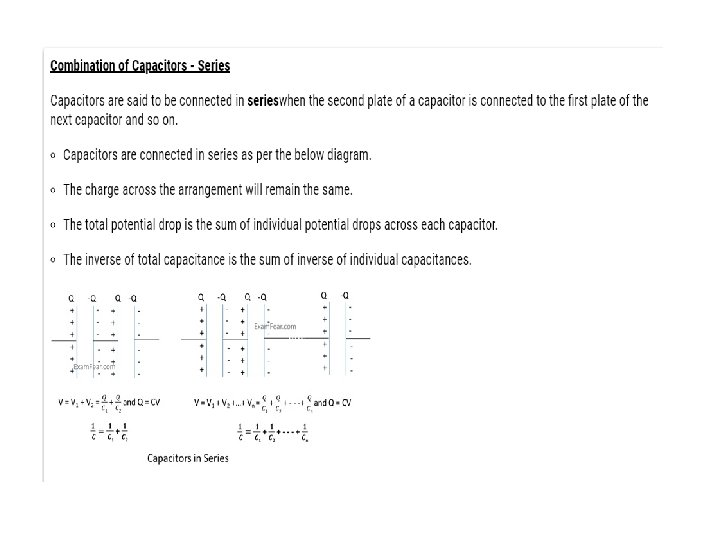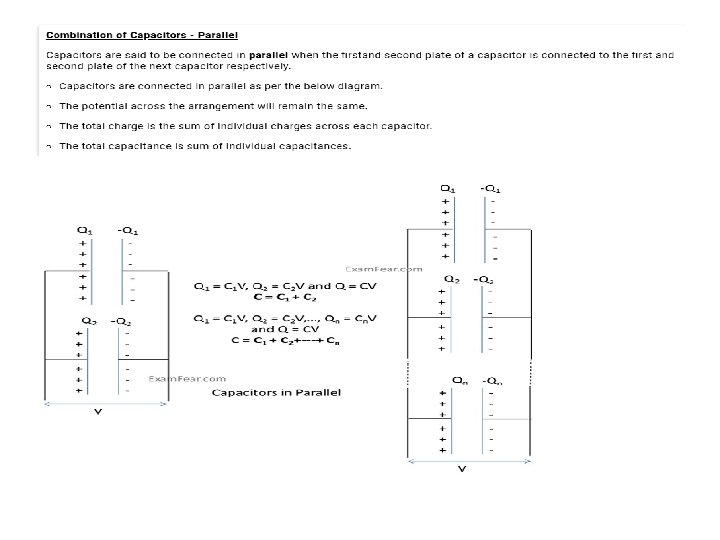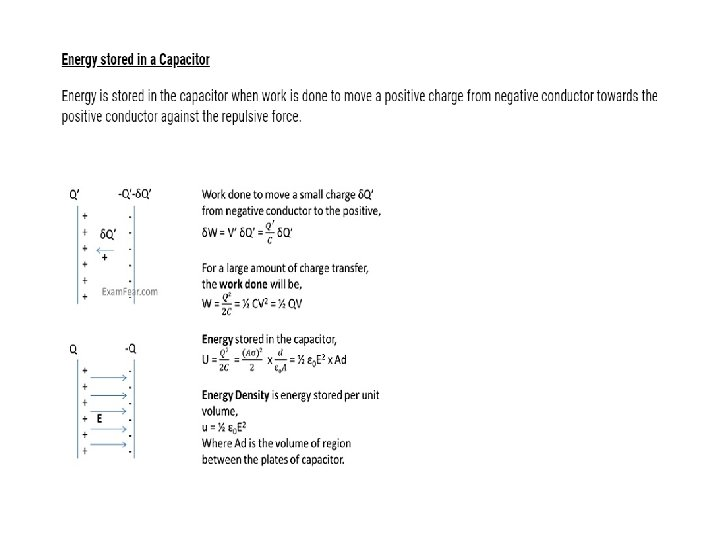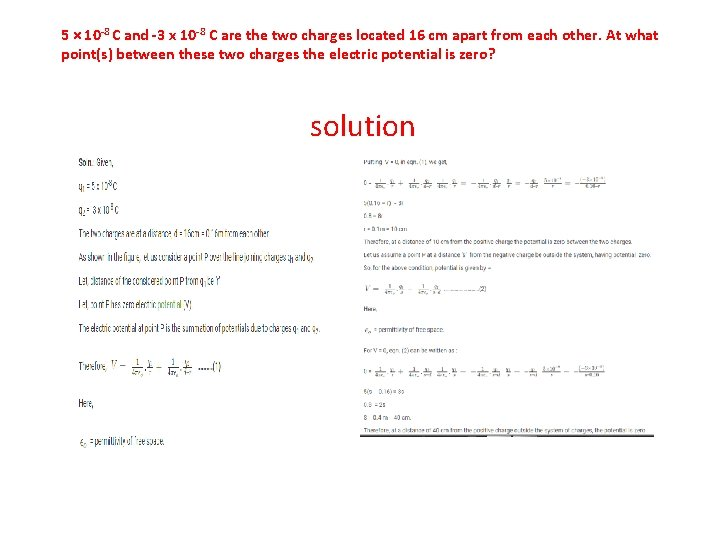5 × 10 -8 C and -3 x 10 -8 C are the two charges located 16 cm apart from each other. At what point(s) between these two charges the electric potential is zero? solutionQ. 2) A regular hexagon having sides equal to 10 cm, contains charges of 5 µC at its each vertices. Find the potential at the centre of this regular hexagon.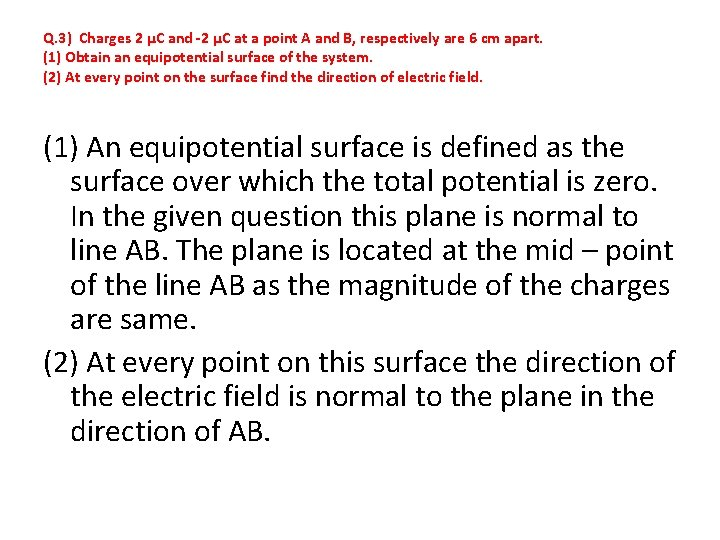Q. 3) Charges 2 µC and -2 µC at a point A and B, respectively are 6 cm apart. (1) Obtain an equipotential surface of the system. (2) At every point on the surface find the direction of electric field. (1) An equipotential surface is defined as the surface over which the total potential is zero. In the given question this plane is normal to line AB. The plane is located at the mid – point of the line AB as the magnitude of the charges are same. (2) At every point on this surface the direction of the electric field is normal to the plane in the direction of AB.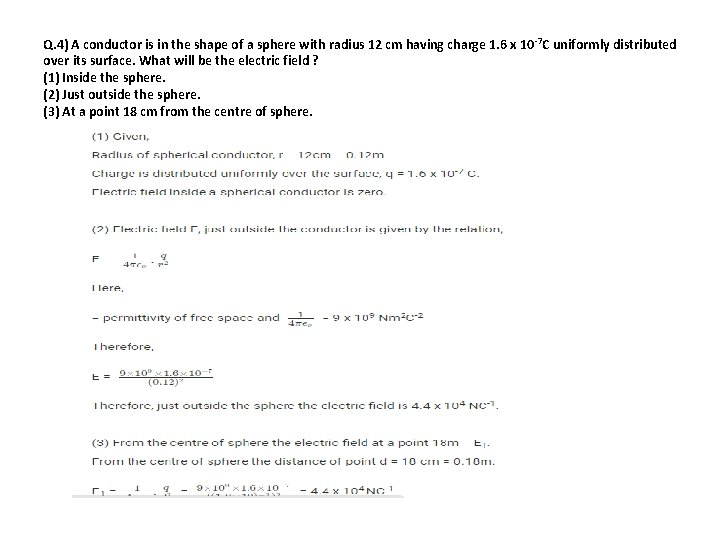Q. 4) A conductor is in the shape of a sphere with radius 12 cm having charge 1. 6 x 10 -7 C uniformly distributed over its surface. What will be the electric field ? (1) Inside the sphere. (2) Just outside the sphere. (3) At a point 18 cm from the centre of sphere.Q. 5) What will be the capacitance of a parallel plate capacitor with air between the plates having capacitance of 8 p. F (1 p. F = 10 -12 F). How much the capacitance of the capacitor will change if the distance between the plate is reduced to half, and the space between the plates is filled with a substance of dielectric constant 6?Q. 6) Three capacitors connected in series have capacitance of 9 p. F each. (1) What can be total capacitance of this combination? (2) Find the potential difference across each capacitor if they are connected to a 120 v supply?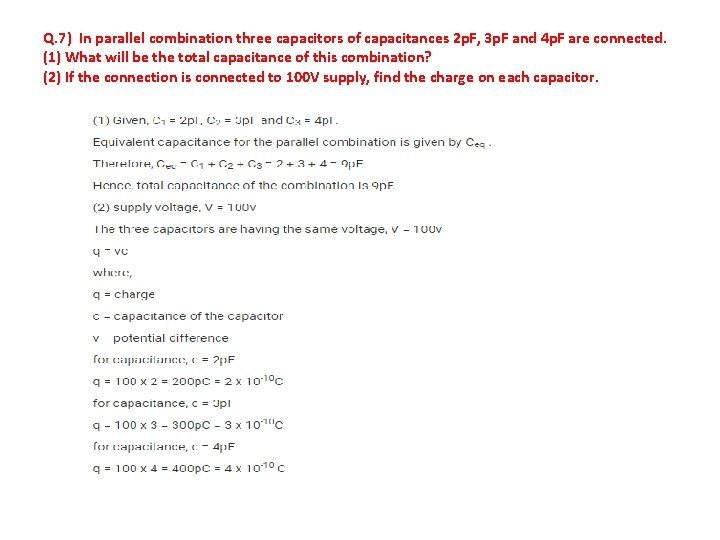Q. 7) In parallel combination three capacitors of capacitances 2 p. F, 3 p. F and 4 p. F are connected. (1) What will be the total capacitance of this combination? (2) If the connection is connected to 100 V supply, find the charge on each capacitor.Q. 8) A parallel plate capacitor having air in between the plates each having area of 6 x 10 3 m 2 and distance between them is 3 mm. Find the capacitance of the capacitor. What will be the charge on each plate if the capacitor is connected to a 100 v source?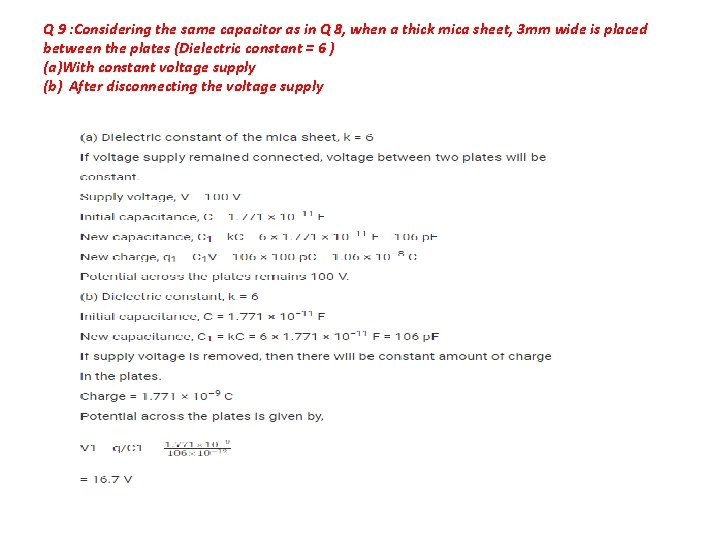Q 9 : Considering the same capacitor as in Q 8, when a thick mica sheet, 3 mm wide is placed between the plates (Dielectric constant = 6 ) (a)With constant voltage supply (b) After disconnecting the voltage supplyQ. 10) How much of electrostatic energy is stored in the capacitor if the capacitor with 12 p. F of capacitance is connected to a 50 v battery?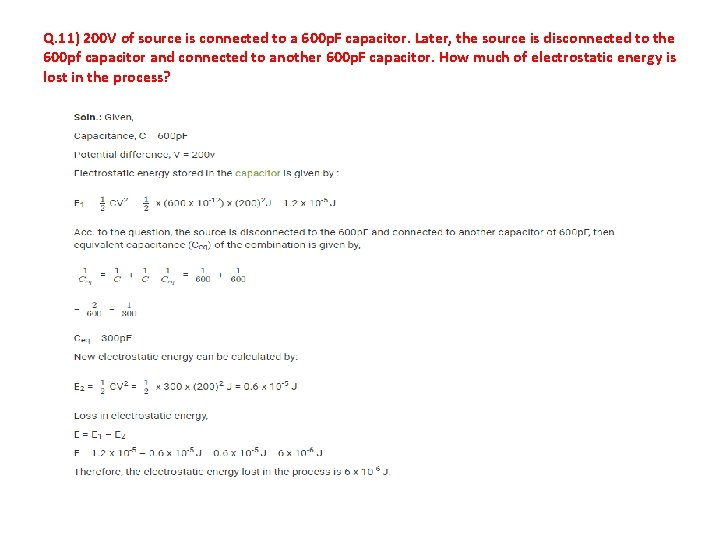Q. 11) 200 V of source is connected to a 600 p. F capacitor. Later, the source is disconnected to the 600 pf capacitor and connected to another 600 p. F capacitor. How much of electrostatic energy is lost in the process?